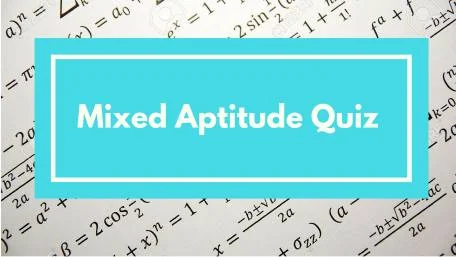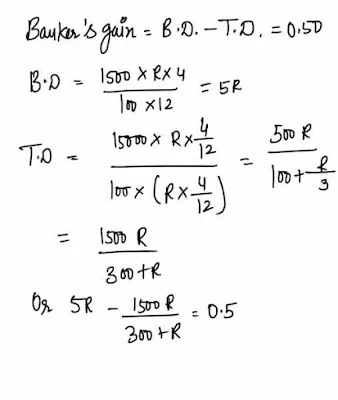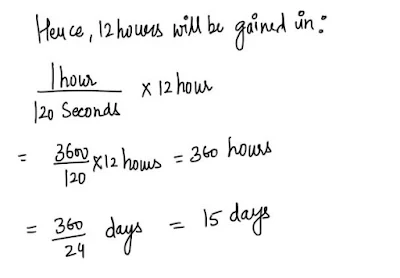New Student User - Use Code HELLO

# Mixed Aptitude Quiz for RBI Grade B - Part 3#### Ques 1.Ans 1.#### Ques 2.

An amount has to be distributed among A, B and C in the ratio 2 : 3: 7 . If A and B together get Rs 1500 less than C, then how much does A get /
(a) 1000
(b) 1500
(c) 2000
(d) None of these
Ans 2. Let a's share , B's share nad C's share  be 2x , 3x and 7x respectively .
2x + 3x = 7x - 1500 =>   x = 750
Hence, A get Rs ( 750 x 2)  i.e.  Rs 1500.

#### Ques 3.

A and B partners contributed capital in the ratio of 4 :5 After three months A and B withdrew 1/4 and 1/5 of their respective capitals. The gain at the end of 10 months was Rs 760. A's share thereof is
(a) Rs 430
(b) rs 330
(c) Rs 550
(d) Rs 400
Ans 3.#### Ques 4.

The average salary of 30 employees in a company is Rs 400.If the manager's salary is included the average becomes Rs 430. What is the manager's salary ?
(a) Rs 1000
(b) Rs 1300
(c) Rs 1200
(d) Rs 1330
Ans 4.Total salary of 30 employees
= Rs ( 400 x 30) = Rs 12000
manager 's salary = Rs ( 430 x 31)
= Rs 13330
Hence, the manager's salary
= Rs ( 13330- 12000) = Rs 1330

#### Ques 5.

If a bill of Rs 1000 is due after 10 years at simple interest of 5% p.a....., What is true discount ?
(a) Rs 1000/3
(b) Rs 1000/7
(c) Rs 250
(d) None of these
Ans 5.#### Ques 6.

The calendar made for the year 1938 did also serve for the year
(a) 1949
(b) 1950
(c) 1951
(d) None of these
Ans 6. Year -->  1938   1939   1940   1941   1942   1943
No. of odd
Days -->                 1         1       2           1         1          1
years   -->          1944   1945   1946     1947    1948
No.of odd
days ---->                 2      1         1           1            2
No. of odd
Total no. of odd days for  these year  = 14
= 0 odd days
Hence, 1949  has the same calendar  as 1938.

#### Ques 7.

The difference between banker's discount and true discount on a bill od rs 1500 fue 4 months hence is 50 paise .Find the approximate rate of simple interest
(a) 5 1/2%
(b) 6 1/2 %
(c) 7 1/2 %
(d) None of these
Ans 7.#### Ques 8.

A wheel, completing 431 revolutions in 4 minutes,makes 2586 revolutions .How many revolutions will be made by another wheel, in the same time as taken by first wheel to make 2586 revolution , completing 329 revolutions in 3 minutes ?
(a) 2632
(b) 3226
(c) 1974
(d) None of these
Ans 8.  Time taken by the first wheel to make
2596 revolutions = 4/431 x 2586 minutes
No. of  revolutions made by the seconds wheel in 24 minutes = 329/3 x 24 = 2632

#### Ques 9.

two watches are together at 12 o'clock. One gains 75 second/hr and the other loses 45 second/hr. When will they together again ?
(a) 15 days
(b) 12 days
(c) 24 days
(d) 11 days'
Ans 9. Time gained by the first clock over the second clock in 1 hour  = 75 + 45 = 120
The first clock must gain  12 hours  over the second clock in  order will  they be together again .#### Ques 10.

If a person wishes to earn 7 1/2 % from his investment , at what price should he buy Rs 500 shares carrying 6% dividend ?
(a) 450
(b) 200
(c) 400
(d) 375
Ans 10.